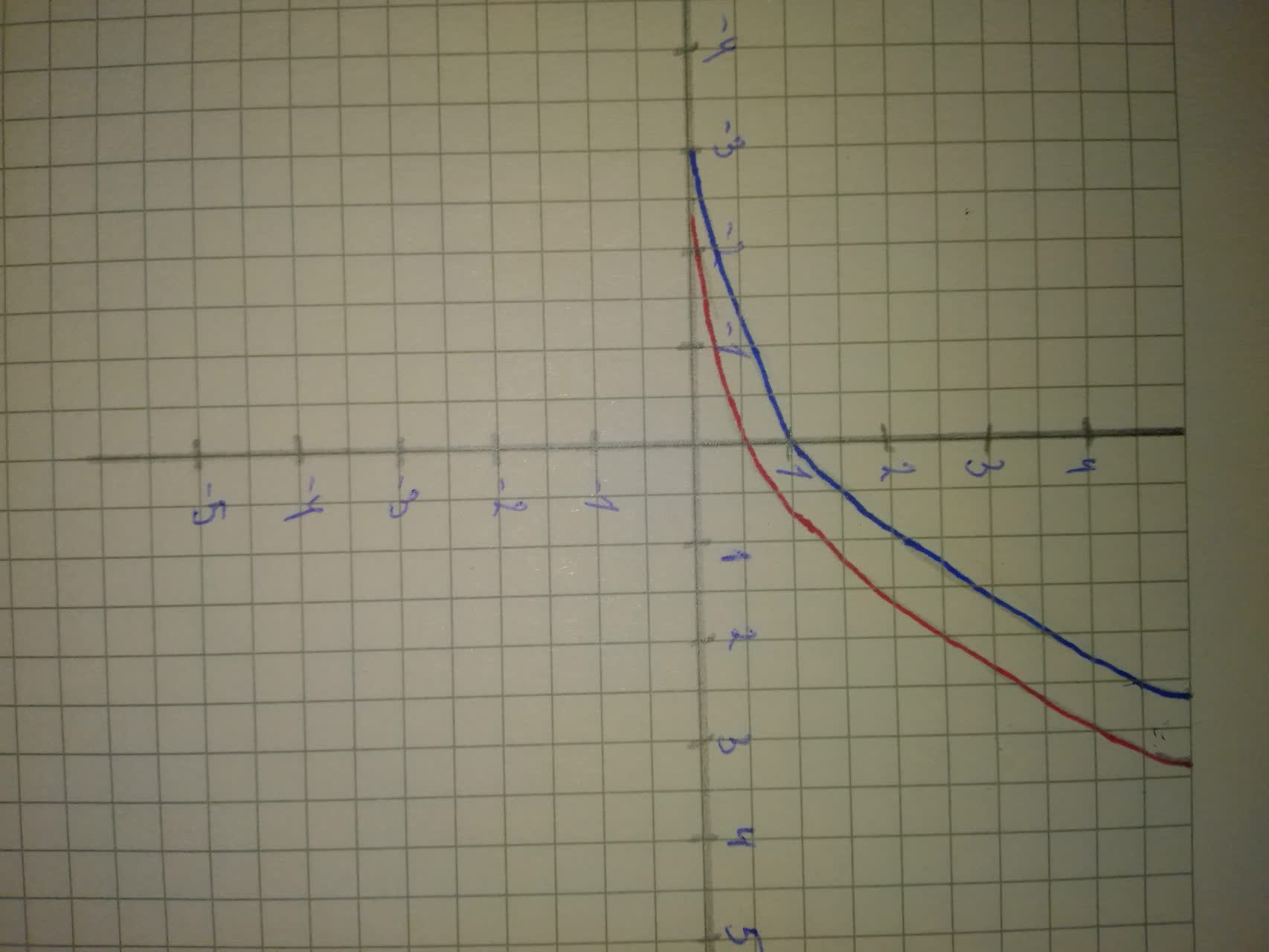Question# Graph and give equations of all asymptotes Use the graphs to determine each function's domain and range f(x)=2^{x}\ and\ g(x)=2^{x-1}

Transformations of functions
ANSWEREDGraph f and g in the same rectangular coordinate system. Use transformations of the graph of f to obtain the graph of g. Graph and give equations of all asymptotes Use the graphs to determine each function's domain and range.
$$\displaystyle{f{{\left({x}\right)}}}={2}^{{{x}}}\ {\quad\text{and}\quad}\ {g{{\left({x}\right)}}}={2}^{{{x}-{1}}}$$Both share a horizontal asymptote $$\displaystyle{y}={0}$$
The domain of both functions is R and range of both functions is $$\displaystyle{\left({0},\infty\right)}$$.